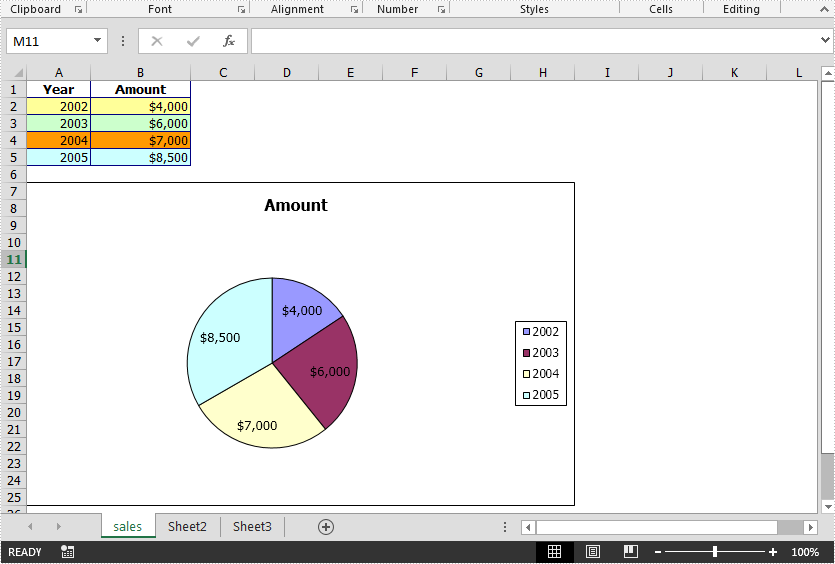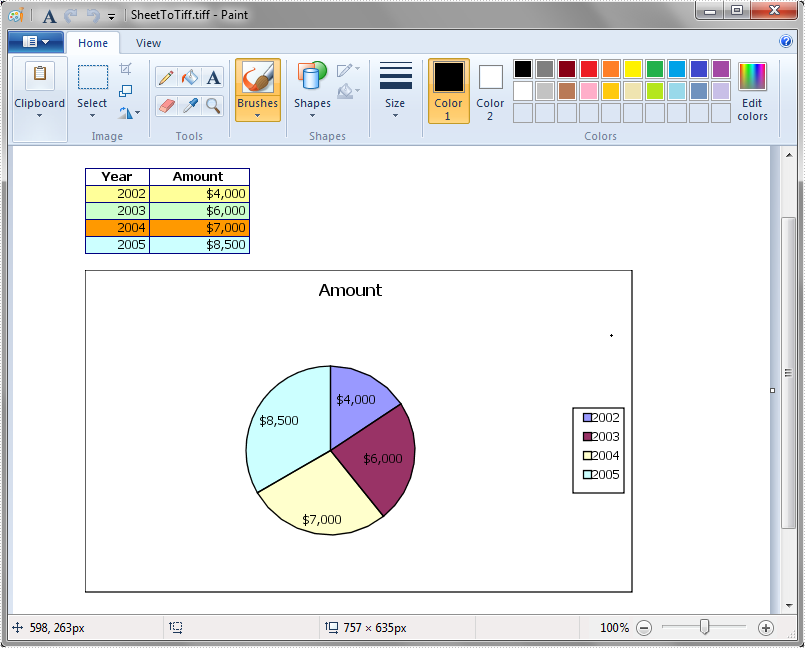Convert Excel to TIFF in Java

This article demonstrates how to convert an Excel worksheet to TIFF and convert a particular cell range in the Excel worksheet to TIFF by using Spire.XLS for Java.

The input Excel file:import com.spire.xls.Workbook;
import com.spire.xls.Worksheet;

public class ConvertExcelToTIFF {
public static void main(String[] args) {
//Create a Workbook instance
Workbook workbook = new Workbook();

//Get the first worksheet
Worksheet sheet = workbook.getWorksheets().get(0);

//Save the first worksheet to TIFF
sheet.saveToTiff("SheetToTiff.tiff");

//Save a particular cell range in the first worksheet to TIFF
//sheet.saveToTiff("CellRangeToTiff.tiff",1,1,5,2);
}
}

The output file after converting the first worksheet to TIFF: